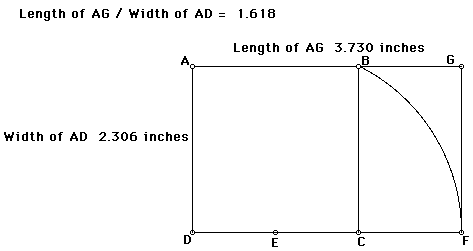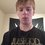# An interesting Number

I found this number quite a while ago just messing around with simple algebra and trigonometry. I am pretty sure it is too simple to have not been found before me, but it has some interesting qualities to it.It is found by constructing the golden rectangle and drawing a line segment to BF and finding its length (this is not the arc illustrated in the image). If the number is 'b': $b = (\sqrt{(5-\sqrt{5})/2}) \approx 1.17557$ There are some relationships between it and the golden ratio of course: $b = \frac{\sqrt{(\varphi x-x)^{2}+x^{2}}}{x}$

$\Phi = \sqrt{(b^{2}-1)}$

$4(b^{2}-1)=(\frac{2}{\varphi})^{2}$ And there is one last relationship which is, at least to me, very interesting. We can represent the golden ratio as $2cos(36^{\circ})$. And what is interesting is that, using simple math, you can get this relationship:

$2\cos(36^\circ) = \frac{\sqrt{5}+1}{2}$

$\cos(36^\circ) = \frac{\sqrt{5}+1}{4}$

$\sin(36^\circ) = \frac{x}{4}$

$4\sin(36^\circ) = 2b$

Finally:

$\boxed{\varphi^{2}+b^{2} = 4}$

Which leads to an interesting question, which I cannot prove, but seems to be the case. It seems that 'b' is the only constant which gives a rational answer to the equation: $\theta = \tan^{-1}\left ( \frac{\varphi}{\sqrt{n^{2}-\varphi^{2}}} \right )$ If theta is measured in degrees and $n \geq 2$. It is also the smallest whole solution to this equation.

Anyway, that is the strange number; I hope it is interesting to someone.Note by Drex Beckman
4 years, 5 months ago

This discussion board is a place to discuss our Daily Challenges and the math and science related to those challenges. Explanations are more than just a solution — they should explain the steps and thinking strategies that you used to obtain the solution. Comments should further the discussion of math and science.

When posting on Brilliant:

• Use the emojis to react to an explanation, whether you're congratulating a job well done , or just really confused .
• Ask specific questions about the challenge or the steps in somebody's explanation. Well-posed questions can add a lot to the discussion, but posting "I don't understand!" doesn't help anyone.
• Try to contribute something new to the discussion, whether it is an extension, generalization or other idea related to the challenge.

MarkdownAppears as
*italics* or _italics_ italics
**bold** or __bold__ bold
- bulleted- list
• bulleted
• list
1. numbered2. list
1. numbered
2. list
Note: you must add a full line of space before and after lists for them to show up correctly
paragraph 1paragraph 2

paragraph 1

paragraph 2

[example link](https://brilliant.org)example link
> This is a quote
This is a quote
    # I indented these lines
# 4 spaces, and now they show
# up as a code block.

print "hello world"
# I indented these lines
# 4 spaces, and now they show
# up as a code block.

print "hello world"
MathAppears as
Remember to wrap math in $$ ... $$ or $ ... $ to ensure proper formatting.
2 \times 3 $2 \times 3$
2^{34} $2^{34}$
a_{i-1} $a_{i-1}$
\frac{2}{3} $\frac{2}{3}$
\sqrt{2} $\sqrt{2}$
\sum_{i=1}^3 $\sum_{i=1}^3$
\sin \theta $\sin \theta$
\boxed{123} $\boxed{123}$

Sort by:

I find this very interesting but I am unable to understand what is $x$.

- 4 years, 4 months ago

Just the length of the square. You can just set them as one. For any real x, you should get the same number.

- 4 years, 4 months ago